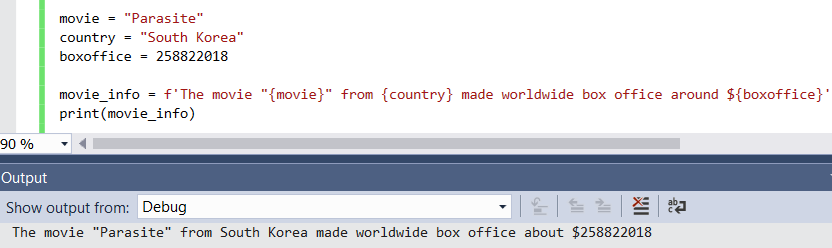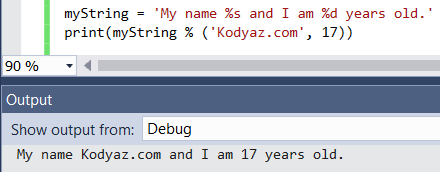﻿ String Format Function with Multiple Variables Python Code SamplePython Programming and Python Code Tutorials Python Programming and Python Code Tutorials for Programmers

# String Format Function with Multiple Variables Python Code Sample

In Python programmers can easily replace variables in a given string value using string format function. I want to show different ways of displaying multiple variables within a string using format function in Python 3 and in Python 2. This Python tutorial includes sample Python code blocks demonstrating use of string format function.

## Display String by Replacing Variables using Python Format String Function

With Python 3, everything can be assumed as objects like strings. Using the format() method of string object, variables with placedholders within the string expression can be evaluated easily.

Here is two ways of displaying string objects with multipe variables using Format function.

In below example, I created a Python function named "compare" which accepts two input variables and compares them arithmetically displaying the comparison result as a single line of string expression.

Following screenshot shows the execution output of the above Python code block on Visual StudioHere is another way of formatting a string value with multiple variable values.
This time the variables are directly referenced within the curly braces {}
This time format function is at the beginning of the string expression

And the output of the above Python code script is seen belowSince there are changes in behaviour of string object between Python version 3 and Python version 2, below code sample code can also help you to format string expressions with variables.

Here is the displayed output after the execution of the sample Python codeI hope Python developers will find useful these hints and sample Python script codes for formatting string expressions including multiple variables.

Python Tutorials